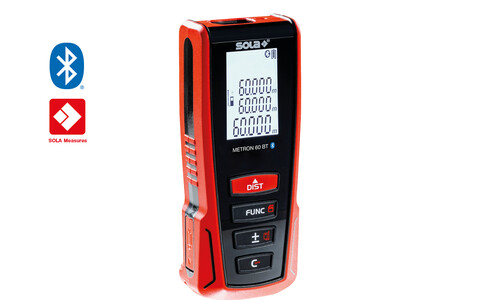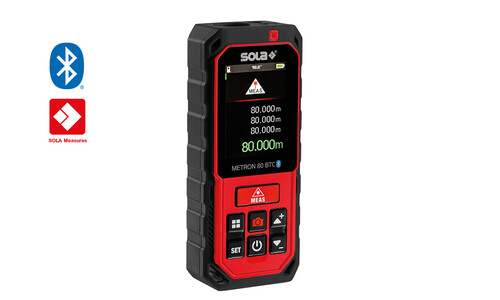# Laser Distance Measurers## WHAT IS A LASER DISTANCE MEASURER?

Laser distance measurer or laser distance meter measures distances between two points with speed and precision. The start point of the measurement is the set measurement edge of the laser distance meter and the second point is the end point of the beam. The maximum range varies depending on the device and can extend to over 100 m. Digital distance measurement offers many advantages over conventional measuring using a folding rule or measuring tape. Distances can be measured precisely and conveniently in just a few seconds. In addition, depending on the model, the devices can calculate areas and volumes of spaces at the touch of a button, function using a touchscreen and have integrated Bluetooth and camera functionalities and an integrated tilt sensor.

## HOW DOES A LASER DISTANCE MEASURER WORK?

The laser focuses on a target for measurement. The distance measurer then emits a laser pulse, which begins the measuring process. The pulse is reflected from the target and received by the photocell in the device. This enables the exact distance to be calculated using the time span between the emission and reflection of the laser. In practice, SOLA uses a process in which the phase change between external pulsing and internal reference pulsing is determined. Only a small surface area is necessary for location when using laser measurement. For ultrasound, it must be much larger in order to enable it to reflect relevant sound waves. Laser distance meters are easier to use and more accurate than ultrasound distance meters, and far more convenient than measuring using a folding rule and measuring tape.

## WHAT MEASURING FUNCTIONS DO LASER DISTANCE MEASURER HAVE?

Laser distance measurer functions vary depending on the manufacturer and device. Conventional devices simply measure distances and show these on the display. But if the user also wants to calculate areas and volumes and determine inaccessible distances or heights via angle measurements, then they should think about purchasing a more complex device. Laser distance meters that have these functionalities are equipped with an integrated tilt sensor. In these times of greater interconnectivity, many laser distance measurers also now come equipped with a Bluetooth interface. Together with the app, lengths can be sketched and dimensioned directly on the smartphone, for example, or measurement results can be saved, managed and shared.### LENGTH MEASUREMENT

Determines a simple distance from the set measurement edge of the laser distance measurer.### VOLUME MEASUREMENT

Automatically calculates an area by measuring the length and width.### VOLUME MEASUREMENT

Calculates the volume of a space by measuring length, width and height.### MIN. / MAX. MEASUREMENT

Determines the minimum or maximum distance by measuring the shortest distance between two points or the greatest distance, e.g. along the diagonal dimension.### CONTINUOUS MEASUREMENT

Rapidly determines a required target distance using continuous measurement.### INDIRECT 3-POINT MEASUREMENT

Determines an inaccessible distance via three length measurements. A measurement is then derived using a 90° angle.### INDIRECT 2-POINT MEASUREMENT

Determines an inaccessible distance via two length measurements. A measurement is then derived using a 90° angle.### INDIRECT MEASUREMENT VIA ANGLE

Measures an indirect and inaccessible height by means of two length measurements. Thanks to the automatic electronic tilt sensor, an additional 90° angle measurement is not required.### INDIRECT DISTANCE MEASUREMENT

Determines an indirect distance without needing to get to the start and end points. Two length measurements are taken from one position to the respective measuring points.### AUTOMATIC DISTANCE MEASUREMENT

Length measurement is used on an elevated target point; its corresponding height and distance are then calculated automatically using the tilt sensor.### TRIANGULAR AREA MEASUREMENT

Calculation of a triangular area by measuring the lengths of the sides.### CIRCULAR AREA MEASUREMENT

Calculation of the circular area by measuring the diameter.### CYLINDER VOLUME MEASUREMENT

Calculation of a cylinder volume by first measuring the diameter of the circular area and then the height of the cylinder.### STAKE OUT FUNCTION

A certain distance is set in the measuring device. The device displays the current distance and starts to beep as you approach the set distance.

Adds and subtracts measurements quickly and simply.

### MEASUREMENT MEMORY

The integrated memory function automatically stores measurements, which can be retrieved at any time.

## WHERE CAN LASER DISTANCE MEASURERS BE USED?

There are a whole range of possible uses for laser distance meters. They are primarily used in the construction industry be it in construction works, renovations, interior design or in exterior works, laser distance meters are needed everywhere. In a matter of seconds, you can calculate distances, areas and volumes of indoor spaces or check measurements and distances. A wide range of occupations use distance meters, ranging from drywall builders, painters, tilers and floorers, joiners and kitchen fitters to carpenters, bricklayers or even architects and interior decorators.

## WHAT ARE THE ADVANTAGES OF A LASER DISTANCE MEASURER?

Laser distance measurers simplify the measuring process, save time and are convenient to use when working. A push of a button is all it takes to set up various measuring functions. Distances can be measured or checked, and areas or volumes calculated in a matter of seconds, all with exceptionally high accuracy, which is far superior to measuring tapes or folding rules. A further advantage of laser distance measurers is that precise measurements can be made alone, without requiring the help of another person. Laser distance meters also make it easier to take measurements in difficult situations, i.e., ‘overhead’ measurements.

## HOW ACCURATE ARE LASER DISTANCE MEASUREMENTS?

The measuring accuracy of laser distance meters varies depending on the device and manufacturer and can usually be found in the technical information or in the operating instructions. Often, laser distance measurers have a measuring accuracy with a tolerance of +/- 2 mm. This measuring tolerance usually refers to a given distance and increases with greater distances. The majority of SOLA laser distance meters have a maximum measuring tolerance of +/- 1.5 mm for distances up to 10 m. The specified measuring accuracy relates to normal measuring conditions. Measurements may become less accurate in adverse conditions, such as direct sunlight, a poorly reflective surface, or measurement on glass or glossy surfaces, leading to measurement errors.

## WHAT RANGE DO LASER DISTANCE MEASURERS HAVE?

As with the measuring accuracy, the maximum range of laser distance measurers varies greatly. Laser distance meters used in the construction industry usually cover measurement distances of 20–60 m.

## OVERVIEW OF SOLA LASER DISTANCE MEASURERS### METRON 30 BT

30 m range, 6 measuring functions, Bluetooth interface for use with the SOLA Measures app, integrated spirit level for pinpoint measurements### METRON 60 BT

60 m range, 9 measuring functions, measured value memory, Bluetooth interface for use with the SOLA Measures app, fold-out support end piece### METRON 80 BTC

80 m range, 16 measuring functions incl. measurement memory, Bluetooth interface for use with the SOLA Measures app, camera function for digital pointfinding (2x and 4x zoom), integrated tilt sensor, timer (self-timer for length measurement), illuminated, rotating color display, protection class IP65### VECTOR 20

20 m range, two measuring functions, developed for quick and easy use### VECTOR 50

50 m range, 7 measuring functions, integrated spirit level for pinpoint measurements